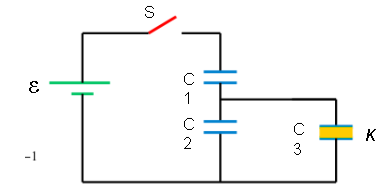# Problem: The switch S is closed for a long time and charges the capacitors. There is a dielectric k in C3. Find the charge and potential on each capacitor.

###### FREE Expert Solution

For capacitors in series, the equivalent capacitance is for two capacitors:

$\overline{){{\mathbf{C}}}_{\mathbf{e}\mathbf{q}}{\mathbf{=}}\frac{{\mathbf{C}}_{\mathbf{1}}{\mathbf{C}}_{\mathbf{2}}}{{\mathbf{C}}_{\mathbf{1}}\mathbf{+}{\mathbf{C}}_{\mathbf{2}}}}$

For capacitors in parallel, the equivalent capacitance is:

$\overline{){{\mathbf{C}}}_{\mathbf{e}\mathbf{q}}{\mathbf{=}}{{\mathbf{C}}}_{{\mathbf{1}}}{\mathbf{+}}{{\mathbf{C}}}_{{\mathbf{2}}}{\mathbf{+}}{\mathbf{.}}{\mathbf{.}}{\mathbf{.}}{\mathbf{+}}{{\mathbf{C}}}_{{\mathbf{n}}}}$

Charge:

$\overline{){\mathbf{Q}}{\mathbf{=}}{\mathbf{C}}{\mathbf{V}}}$

C2 and C3 are in parallel.

C23 = C2 + kC3

C1 and C23 are in series.

C123 = C1(C2 + kC3)/(C1 + C2 + kC3)

100% (118 ratings)###### Problem Details

The switch S is closed for a long time and charges the capacitors. There is a dielectric k in C3. Find the charge and potential on each capacitor.